### Home > A2C > Chapter 10 > Lesson 10.2.3 > Problem10-95

10-95.
1. In the card game called “Twenty-One,” two cards are dealt from a randomly shuffled deck (a regular deck of 52 cards). The player's goal is to get the sum of his or her cards to be as close to 21 as possible, without going over 21. To establish the sample space for this problem, you need to think of choosing two from a set of 52. Homework Help ✎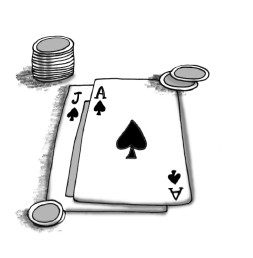1. How many ways are there to do this?

2. The tens, jacks, queens, and kings all have a value of 10 points. There are four of each in a standard deck. How many ways are there to choose two cards with a value of 10 out of the 16 that are in the deck? What is the probability of being dealt two ten-valued cards?

3. If you did not already do so, write your solution to part (b) in the form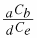.

4. What is the probability of being dealt two face cards? (Face cards are Kings, Queens and Jacks, the cards that have faces.)

5. If you did not already do so, write your solution to part (d) in the form.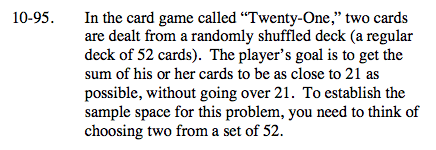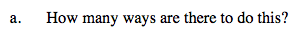$_{n}C_{r}=\frac{n!}{r!(n-r!)}$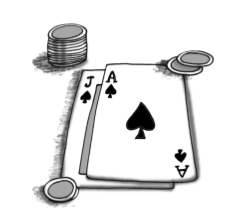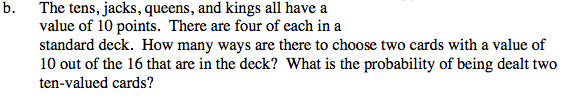How many ways are there to select two cards from the set of ten-valued cards?

Divide this number by the total number of 2-card hands.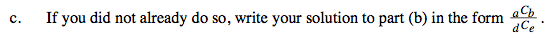$\frac{_{2}C_{1}6}{_{2}C_{5}2}$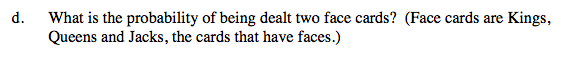See part (b).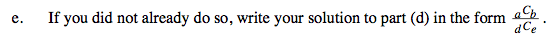See part (c).